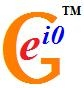# User blogs

Tag search results for: "pheoretical physicist"Physics as Infinite-dimensional Geometry IV: Weak Form of Electric-Magnetic Duality and Its Implications (by Matti Pitkänen): Abstract: The notion of electric-magnetic duality emerged already two decades ago in the attempts to formulate the Kähler geometry of the "world of classical worlds". Quite recently a considerable step of progress took place in the understanding of this notion. This concept leads to the identification of the physical particles as string like objects defined by magnetic charged wormhole throats connected by magnetic flux tubes. The second end of the string contains particle having electroweak isospin neutralizing that of elementary fermion and the size scale of the string is electro-weak scale would be in question. Hence the screening of electro-weak force takes place via weak confinement. This picture generalizes to magnetic color confinement. Electric-magnetic duality leads also to a detailed understanding of how TGD reduces to almost topological quantum field theory. The condition that the theory reduces to almost topological QFT and the hydrodynamical character of field equations leads to a detailed ansatz for the general solution of field equations and also for the solutions of the modified Dirac equation relying on the notion of Beltrami flow for which the flow parameter associated with the flow lines defined by a conserved current extends to a global coordinate. This makes the theory is in well-defined sense completely integrable. Also Dirac determinant conjectured to represent Kähler function can be calculated explicitly in terms of the geometric data characterizing 3-surfaces. http://prespacetime.com/index.php/pst/article/view/51

Physics as Generalized Number Theory I: p-Adic Physics and Number Theoretic Universality (by Matti Pitkänen)

Physics as Generalized Number Theory II: Classical Number Fields (by Matti Pitkänen)

Abstract: Physics as a generalized number theory program involves three threads: various p-adic physics and their fusion together with real number based physics to a larger structure, the attempt to understand basic physics in terms of classical number fields discussed in this article, and infinite primes whose construction is formally analogous to a repeated second quantization of an arithmetic quantum field theory. In this article the connection between standard model symmetries and classical number fields is discussed. The basis vision is that the geometry of the infinite-dimensional WCW ("world of classical worlds") is unique from its mere existence. This leads to its identification as union of symmetric spaces whose Kähler geometries are fixed by generalized conformal symmetries. This fixes space-time dimension and the decomposition M4 X S and the idea is that the symmetries of the Kähler manifold S make it somehow unique. The motivating observations are that the dimensions of classical number fields are the dimensions of partonic 2-surfaces, space-time surfaces, and imbedding space and M8 can be identified as hyper-octonions- a sub-space of complexified octonions obtained by adding a commuting imaginary unit. This stimulates some questions. http://prespacetime.com/index.php/pst/article/view/53

Physics as Generalized Number Theory III: Innite Primes (by Matti Pitkänen)

Abstract: Physics as a generalized number theory program involves three threads: various p-adic physics and their fusion together with real number based physics to a larger structure, the attempt to understand basic physics in terms of classical number fields, and infinite primes discussed in this article. The construction of infinite primes is formally analogous to a repeated second quantization of an arithmetic quantum field theory by taking the many particle states of previous level elementary particles at the new level. Besides free many particle states also the analogs of bound states appear. In the representation in terms of polynomials the free states correspond to products of first order polynomials with rational zeros. Bound states correspond to n-th order polynomials with non-rational but algebraic zeros. The construction can be generalized to classical number fields and their complexifications obtained by adding a commuting imaginary unit. Special class corresponds to hyper-octonionic primes for which the imaginary part of ordinary octonion is multiplied by the commuting imaginary unit so that one obtains a sub-space M8 with Minkowski signature of metric. Also in this case the basic construction reduces to that for rational or complex rational primes and more complex primes are obtained by acting using elements of the octonionic automorphism group which preserve the complex octonionic integer property. http://prespacetime.com/index.php/pst/article/view/55

The Geometry of CP2 and its Relationship to Standard Model (by Matti Pitkänen)

Abstract: This appendix contains basic facts about CP2 as a symmetric space and Kähler manifold. The coding of the standard model symmetries to the geometry of CP2, the physical interpretation of the induced spinor connection in terms of electro-weak gauge potentials, and basic facts about induced gauge fields are discussed. http://prespacetime.com/index.php/pst/article/view/56

· Apr 11 '12 ·